# Conditionals

When you write a computer program the commands or statements which you write in the chosen programming language are listed in order one after the other in the source code file. The order in which these statements are executed when the program is running however is usually not the same. Controlling which statements in a program are executed and the order in which they are executed is called Flow of Control.

Frequently in a program you will want to test whether a particular condition is true for false. For example, does a variable hold a particular value, or has some event occurred, etc. This can be outlined in pseudocode as:

```
if(some condition is true) then
do this
else

```

### The bool data type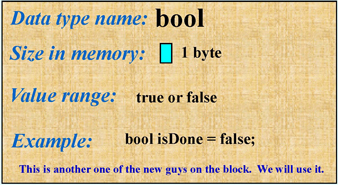You should already be familiar with the bool data type. In a program you an create variables of type bool such as
```
bool isDataOK;
bool done;

```
and set these variables to either true (isDataOK = true;) or false (done = false;). The state or condition of many other values can also be evaluated as either true or false. These conditions can be tested using a set of Relational Operators to evaluate what are called Logical Expressions.

### Logical Expressions

These state a condition which can be either true or false.
• bool isDataOK; – Boolean expression which can be true or false.
• (x < y) – Is x less than y? If x and y are int variables this condition can be true or false.
• (a < b) AND (c > d) – Two logical expressions. If a, b, c and d are int variables this condition can be true (if a is less than b and c is greater than d) or false.

### Relational Operators

In C/C++ we have these relational operators which can be used in conditional expressions:
 == Equal to (be careful with this one. Remember two '=' characters) != Not equal < Less than > Greater than <= Less than or equal (note, you cannot say =<) >= Greater than or equal (note, you cannot say =>)
Examples

Assume you have the following variables declared and initialized:
```	int x = 10;
int y = 15;
char ch1 = 'm';
char ch2 = 'n';
float f1 = 1.2345;
float f2 = 1.2345;
string s1 = "This is a string";
string s2 = "This is a string, too";
```
The following table shows some conditional statements and what their "value" (true or false) state will be:
 (x < y) is true (ch1 < ch2) is true (f1 < f2) is false (x == y) is false (ch1 > ch2) is false (f1 == f2) is true (maybe, see warning below) (x <= y) is true (ch1 >= ch2) is false (f1 != f2) is false (maybe, see warning below) (x == y) is false (ch1 != ch2) is true (f1 >= f2) is true (maybe, see warning below) (s1 < s2) is true. Operators are overloaded for strings. Less than means comes before in alphabetical order (s1 != s2) is true.

Warning
1. Don’t try to compare floats or doubles as the internal representation of these can make two seemingly equal values appear not equal.
2. If you compare a Boolean with an int (example; (isDataOK < 5)) the boolean value true is type coerced to the integer value 1 and the boolean value false is type coerced to the integer value 0. That means this conditional will always be false since both 0 and 1 are less than 5.

## The if statement

Now we come to where we combine conditional statements with flow of control. The if statement is used to test a conditional expression and execute one or more statements if that conditional is true. The general form of the if statement looks like this: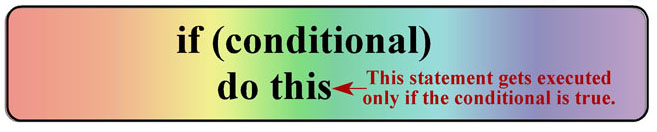If the conditional expression is true then the line of code immediately after the if statement will be executed. If the conditional is not true then the line of code immediately after the if statement will not be executed and "flow of control" will pass to the next statement.

## The if-else statement

You can also provide an alternate line of code to be executed if the conditional statement is false. The general form of the if-else statement looks like this: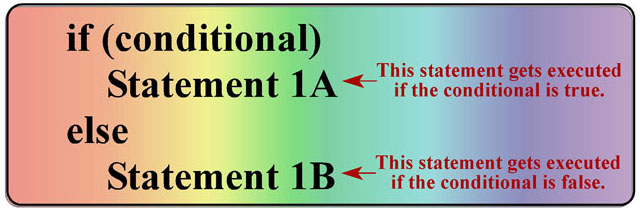If the conditional expression is true then the line of code immediately after the if statement will be executed. If the conditional is not true then the line of code immediately after the else statement will be executed and then "flow of control" will pass to the next statement following the if-else statement.

Executing more than one line of code after an if or else statement
Most of the time when you use an if or an if-else statement in a program you will want to exeute more than just one line of code depending on the state of the conditional. To do this use braces to enclose the block of code to be exeuted for the if part of the statement an for the else part of the statement.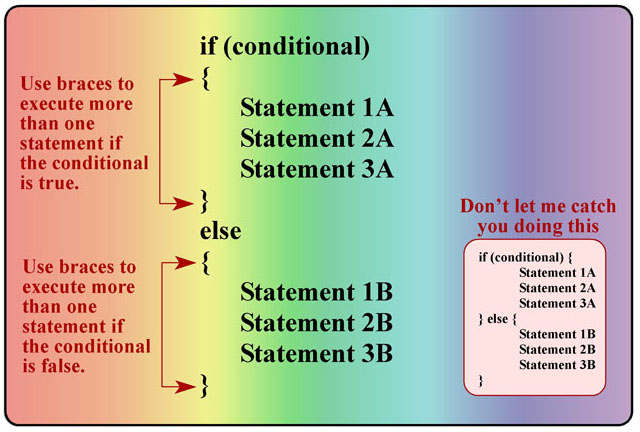## Warning

This is a common error. Look at the code below:
```	int n;
cin >> n;
if(n = 3)
cout << "n equals 3" << endl;
else
cout << "n not equal 3" << endl;
```
This code ALWAYS prints "n equals 3". Did you expect it to only print "n equals 3" when the user inputs the value 3? Notice that the conditional expression is (n = 3) not (n == 3). Here is what actually happens when you do this. Note: this says WHEN you do this, not IF you ever do this, because you will!:
1. At the statement cin >> n; the user will input an integer value.
2. The statement in parentheses is NOT a conditional statement. It actualls sets the variable n equal to 3.
3. Now with n equal to 3 the actual conditional that is tested is if (n). But, If n is zero the conditional is evaluated as false. If n is not zero the conditional is evaluated as true.

### Nested if statements

It is possible to "string" several if statements together to provide multiple branching flow of control statements. Look at the general format of a nested if statement below: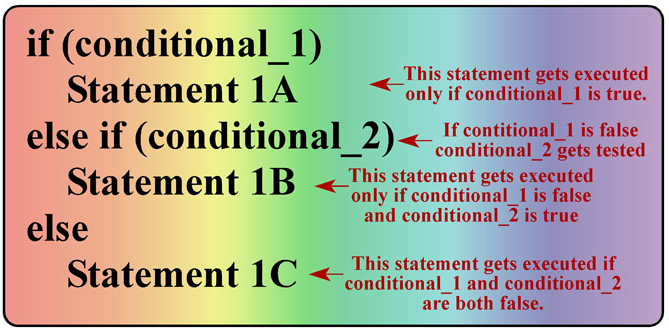This means you can include commands like the one below in your code:
```
if (x < y)
cout << "x is less than y" << endl;
else if (x == y)
cout << "x is equal to y"  << endl;
else
cout << "x is greater than y" << endl;
```

## Warning: The dangling else

There is something else to be careful not to do. Look at the code below. What we want to test is if average is between 60 and 70 then print "Passing but marginal" else print "Failing".
```	if (average >= 60)
if(average < 70)
cout << "Passing but marginal";
else
cout << "Failing";
```

What we will actually get is, if average is less than 60 the message "Failing" will never be printed. In spite of the fact that we have formatted the statement to look like the else is paired with if (average >= 60) it is actually paired with if(average < 70). An else is always paired with the closest if unless braces are used to modify this. To correct this code so that the else is actually paired with if (average >= 60) add the braces as shown below:
```	if (average >= 60)
{
if(average < 70)
cout << "Passing but marginal";
}
else
cout << "Failing";
```
Now it will work correctly.

### Logical Operators

Sometimes you want to check more than one condition in the same statement. For example:
```
If (this is true) AND (that is true)
If (this is true) OR (that is true)
If NOT (this is false)
```

C/C++ prlvides three logical operators to let you do this. These are:
• && - Logical AND
• || - Logical OR
• ! - Logical NOT

Look at these examples:
```	if ((x == 5) && (y < 10))
cout << "both conditions OK" << endl;
```
In this example if x is equal to 5 AND y is less than 10 then the string "both conditions OK" will be printed.
```if ((x == 5) || (y < 10))
cout << "At least one condition is OK" << endl;
```
In this example if x is equal to 5 OR y is less than 10 then the string "At least one condition is OK" will be printed.
```if (!(x == y))
cout << "x not equal to y" << endl;
```
In this example if x is NOT equal to y then the string "At least one condition is OK" will be printed. Note that the conditional (!(x == y)) is actually the same as (x != y).

One more thing to be aware of in these examples of using logial operators. The actual evaluation of the conditional statements follows what is known as short-circuit evaluations.
```
if(condition 1) && (condition 2)
```

If condition 1 is false then condition 2 is never evaluated. It doesn't matter the state of condition 2. If the first condition is false then the whole statement will be false.
```
if(condition 1) || (condition 2)
```
If condition 1 is true then condition 2 is never evaluated because there is no need to do so. The whole statement will already be considered true.

The following truth tables summarize this: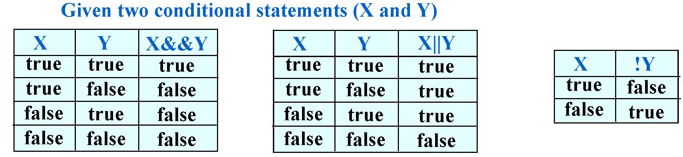### Precedence with Conditional Operators

The precedence (order of execution in a mixed expression) is determined by the following table. Unary operators group from right to left (unary + - !). Binary operators group left to right (math and other logical operators).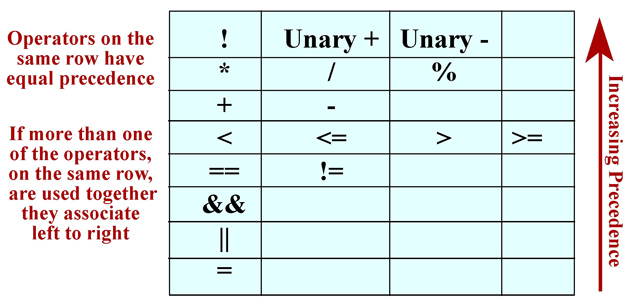### Testing the state of an I/O Stream

Look at code in red below. You can test an ifstream or ofstream object at any time to make sure there has not been an error and the read was good by calling the good() function of the ifstream or ofstream object. Note that the author of the textbook says to do this test with this code: if(!inFile). Professional software engineers do not do it this way. Use the fstream function good() to test if the last action on the file was successful.
```	#include <iostream>
#include <fstream>
#include <string>
using namespace std;

int main()
{
string inFileName, outFileName;
ifstream inFile;
ofstream outFile;
int year, month, day;
string str1, str2;
// Prompt user for input
cout << "Enter the name of the input data file." << endl;
cin >> inFileName;
cout << "Enter the name of the output file." << endl;
cin >> outFileName;
// Let user know what is happening
cout << "Opening data file " << inFileName << " for input." << endl;
inFile.open(inFileName.c_str());

if(!inFile.good())
{
cout << "Failed to open data file. Program terminating." << endl;
return 0;
}

cout << "Opening data file " << outFileName << " for output." << endl;
outFile.open(outFileName.c_str());

if(!outFile.good())
{
cout << "Failed to ouput data file. Program terminating." << endl;
return 0;
}
// The rest of the input code section of this example is here.
}
```

### Conditional expressions

In some situations a simple if-else statement can be replaced with a conditional expression. The syntax of a conditional expression consists of a conditional statement in parentheses. This can be just like the conditionals used in an if-else statement. This is followed by a question mark, a value to be set if the condition is true, a colon, and finally a value to be set if the condition is false.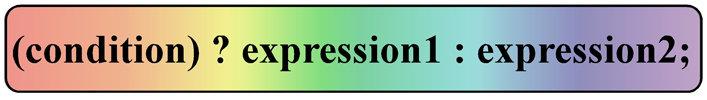Suppose you have this if-else statement:
```	if(a < b)
max = a;
else
max = b;
```
This if-else expression can also be written using the conditional expression syntax:
```	max = (a < b) ? a : b;
```
The conditional expression is handy short-hand syntax for certain situations, but be careful, you cannot substitued a conditional expression for every if-else statement.

### The Switch statement

In some situations where you have a nested if it may be more efficient to use a switch statement. The general syntax for a switch statement is: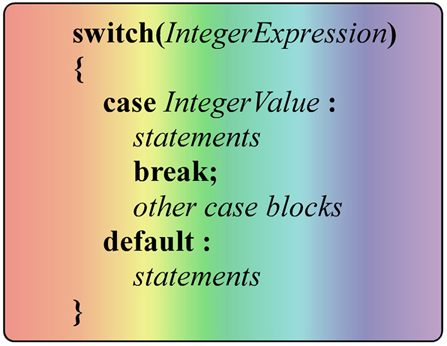This consists of the keyword switch followed by an interger expression (usually just an integer variable) in parentheses. Then enclosed in braces will be any number of case blocks consisting of the keyword case, a value which the interger expression could take and a colon. This is followed by any number of statements to be executed if the interger expression has this value. The list of statements to be excuted is then terminated using the break keyword. You may also end the list of case blocks with an optional default block containing statements to be executed if the integer expression holds none of the given case values.

Suppose you have the following nexted if-else statement:
```	int dNum;
cout << "Which doctor do you want to see?\n"
cout << "(1)Smith, (2)Jones, (3)Quack\n";
cin >> dNum;

if(dNum == 1)
cout << "Down the hall to room 123\n";
else if(dNum == 2)
cout << "Up stairs to room 234\n";
else if(dNum == 3)
cout << "Take elevator to room 1313\n";
else
cout << "Sorry, no such doc here.\n';
```
This can be rewritten in the form of a switch statement:
```	int dNum;
cout << "Which doctor do you want to see?\n"
cout << "(1)Smith, (2)Jones, (3)Quack\n";
cin >> dNum;

switch(dNum)
{
case 1 : cout << "Down the hall to room 123\n";
break;
case 2 : cout << "Up stairs to room 234\n";
break;
case 3 : cout << "Take elevator to room 1313\n";
break;
default: cout << "Sorry, no such doc here.\n";
}
```
Here is an example of using a swith statement with a char variable as the interger expression.
```	char grade;
// Variable grade set to 'A', 'B', 'C', 'D', or 'F'

{
case 'A' : cout << "Excellent work\n";
break;
case 'B' : cout << "Good work\n";
break;
case 'C' : cout << "Average work\n";
break;
case 'D' : cout << "Poor work\n";
break;
case 'F' : cout << "Change your major!\n";
break;
}
```
If you fail to include the break statement at the end of the list of statements to be executed for a case block then execution will "fall through" to the next case block and execute the statements there. Sometimes this may be what you want. Suppose in the example above the char variable grade could hold either an upper or lower case letter grade. The following switch statement would give the appropriate message regardless of the case of the leter grade.
```	char grade;
// Variable grade set to 'a', 'A', 'b', 'B', 'c', 'C', 'd', 'D', 'f', or 'F'

{
case 'a' : // No break so execution falls through to the next case
case 'A' : cout << "Excellent work\n";
break;
case 'b' :
case 'B' : cout << "Good work\n";
break;
case 'c' :
case 'C' : cout << "Average work\n";
break;
case 'd' :
case 'D' : cout << "Poor work\n";
break;
case 'f' :
case 'F' : cout << "Change your major!\n";
break;
}
```